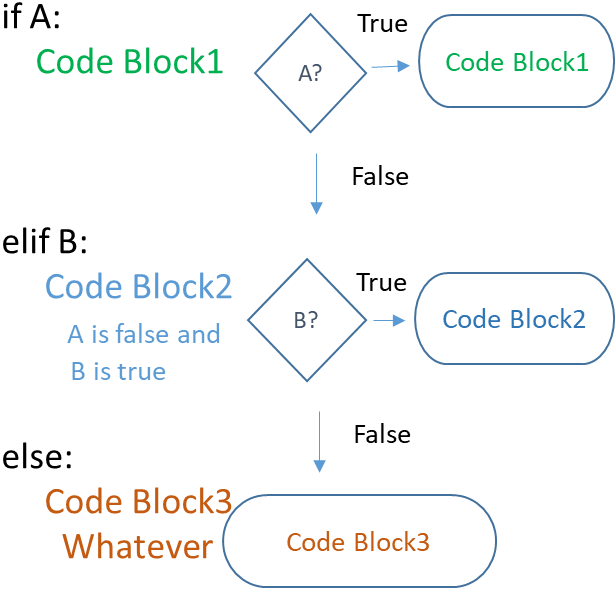# grade in exam using if else elif in Python

Mark of a student in Exam is taking as input. Based on this mark grade is decided by using if , elif and else.

More than or equal to 80 is A grade
More than or equal to 60 is B grade
More than or equal to 40 is C grade
Less than 40 mark is Fail
``````m=int(input("Enter your mark "))
if(m>=80):
elif(m>=60):
elif(m>=40):
else:
print("Failed in this Exam ")``````Python if else and elif to execute code blocks based on condition checking True or False

Subscribe to our YouTube Channel here

## Subscribe

* indicates required
Subscribe to plus2netplus2net.com